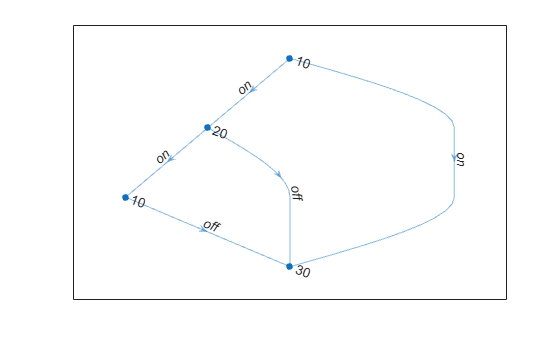# Add Graph Node Names, Edge Weights, and Other Attributes

This example shows how to add attributes to the nodes and edges in graphs created using `graph` and `digraph`. You can specify node names or edge weights when you originally call `graph` or `digraph` to create a graph. However, this example shows how to add attributes to a graph after it has been created.

### Create Graph

Create a directed graph. The corresponding elements in `s` and `t` define the source and target nodes of each edge in the graph.

```s = [1 1 2 2 3]; t = [2 4 3 4 4]; G = digraph(s,t)```
```G = digraph with properties: Edges: [5x1 table] Nodes: [4x0 table] ```

Add node names to the graph by adding the variable, `Name`, to the `G.Nodes` table. The `Name` variable must be specified as an `N`-by-`1` cell array of character vectors or string array, where `N = numnodes(G)`. It is important to use the `Name` variable when adding node names, as this variable name is treated specially by some graph functions.

`G.Nodes.Name = {'First' 'Second' 'Third' 'Fourth'}';`

View the new `Nodes` table.

`G.Nodes`
```ans=4×1 table Name __________ {'First' } {'Second'} {'Third' } {'Fourth'} ```

Use table indexing to view the names of nodes 1 and 4.

`G.Nodes.Name([1 4])`
```ans = 2x1 cell {'First' } {'Fourth'} ```

Add edge weights to the graph by adding the variable, `Weight`, to the `G.Edges` table. The `Weight` variable must be an `M`-by-1 numeric vector, where `M = numedges(G)`. It is important to use the `Weight` variable when adding edge weights, as this variable name is treated specially by some graph functions.

`G.Edges.Weight = [10 20 30 40 50]';`

View the new `Edges` table.

`G.Edges`
```ans=5×2 table EndNodes Weight ________________________ ______ {'First' } {'Second'} 10 {'First' } {'Fourth'} 20 {'Second'} {'Third' } 30 {'Second'} {'Fourth'} 40 {'Third' } {'Fourth'} 50 ```

Use table indexing to view the first and third rows of `G.Edges`.

`G.Edges([1 3],:)`
```ans=2×2 table EndNodes Weight ________________________ ______ {'First' } {'Second'} 10 {'Second'} {'Third' } 30 ```

In principle you can add any variable to `G.Nodes` and `G.Edges` that defines an attribute of the graph nodes or edges. Adding custom attributes can be useful, since functions like `subgraph` and `reordernodes` preserve the graph attributes.

For example, add a variable named `Power` to `G.Edges` to indicate whether each edge is `'on'` or `'off'`.

```G.Edges.Power = {'on' 'on' 'on' 'off' 'off'}'; G.Edges```
```ans=5×3 table EndNodes Weight Power ________________________ ______ _______ {'First' } {'Second'} 10 {'on' } {'First' } {'Fourth'} 20 {'on' } {'Second'} {'Third' } 30 {'on' } {'Second'} {'Fourth'} 40 {'off'} {'Third' } {'Fourth'} 50 {'off'} ```

Add a variable named `Size` to `G.Nodes` to indicate the physical size of each node.

```G.Nodes.Size = [10 20 10 30]'; G.Nodes```
```ans=4×2 table Name Size __________ ____ {'First' } 10 {'Second'} 20 {'Third' } 10 {'Fourth'} 30 ```

### Modify Tables with Variables Editor

Since `Nodes` and `Edges` are both tables, you can use the Variables editor to interactively view or edit the tables. For more information, see Create and Edit Variables.

### Label Nodes and Edges of Graph Plot

When you plot a graph, you can use the variables in `G.Nodes` and `G.Edges` to label the graph nodes and edges. This practice is convenient, since these variables are already guaranteed to have the correct number of elements.

Plot the graph and label the edges using the `Power` variable in `G.Edges`. Label the nodes using the `Size` variable in `G.Nodes`.

`p = plot(G,'EdgeLabel',G.Edges.Power,'NodeLabel',G.Nodes.Size)````p = GraphPlot with properties: NodeColor: [0 0.4470 0.7410] MarkerSize: 4 Marker: 'o' EdgeColor: [0 0.4470 0.7410] LineWidth: 0.5000 LineStyle: '-' NodeLabel: {'10' '20' '10' '30'} EdgeLabel: {'on' 'on' 'on' 'off' 'off'} XData: [2 1.5000 1 2] YData: [4 3 2 1] ZData: [0 0 0 0] Use GET to show all properties ```